### Home > INT3 > Chapter 12 > Lesson 12.2.3 > Problem12-114

12-114.
1. You have a bag with 60 black jellybeans and 240 red jellybeans. Homework Help ✎

1. If you draw one jellybean out of the bag, what is the probability that it is black?

2. If you add 60 black jellybeans to the original bag and draw one jellybean, what is the probability that it is black?

3. How many black jellybeans do you need to add to the original bag to double the original probability of drawing one black jellybean?

4. Write an equation that represents the problem in part (c).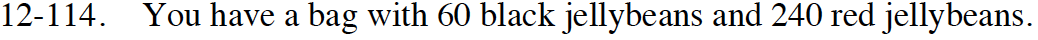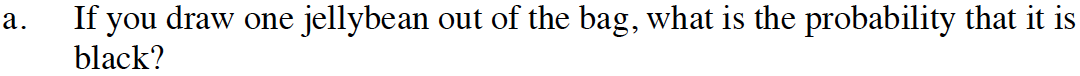$\frac{60}{60+240}=?$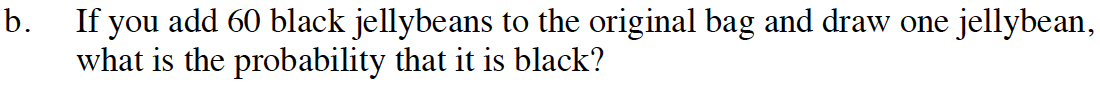$\frac{60+60}{60+60+240}=?$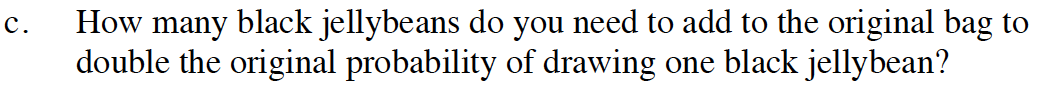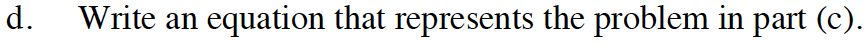$\frac{60+x}{60+x+240}=2(\text{the answer from part(a)})$